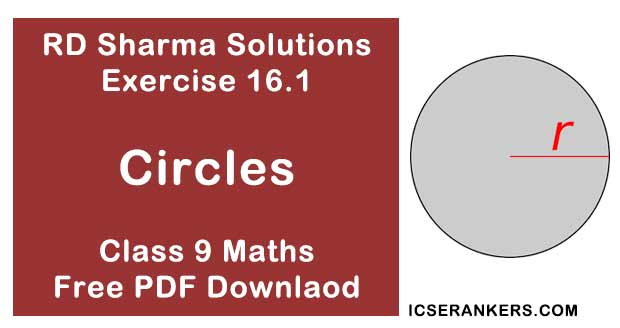# Chapter 16 Circles RD Sharma Solutions Exercise 16.1 Class 9 MathsChapter Name RD Sharma Chapter 16 Circles Exercise 16.1 Book Name RD Sharma Mathematics for Class 10 Other Exercises Exercise 16.2Exercise 16.3Exercise 16.4Exercise 16.5 Related Study NCERT Solutions for Class 10 Maths

### Exercise 16.1 Solutions

1. Fill in the blanks :
(i) All points lying inside/outside a circle are are called .... points/ .... points.
(ii) Circles having the same centre and different radii are called .... circles.
(iii) A point whose distance from the centre of a circle is greater than its radius lies in .... of the circle.
(iv) A continuous piece of a circle is .... of the circle.
(v) The longest chord of a circle is a . . . . of the circle.
(vi) An arc is a . . . . when its ends are the ends of a diameter.
(vii) Segment of a circle is the region between an arc and . . . . of the circle.
(viii) A circle divides the plane, on which it lies, in . .. parts.

Solution

(i) Interior/exterior
(ii) Concentric
(iii) The exterior
(iv) Arc
(v) Diameter
(vi) Semi-circle
(vii) Centre
(viii) Three

2. Write the truth value (T/F) of the following with suitable reasons:
(i) A circle is a plane figure.
(ii) Line segment joining the centre to any point on the circle is a radius of the circle,
(iii) If a circle is divided into three equal arcs each is a major arc.
(iv) A circle has only finite number of equal chords.
(v) A chord of a circle, which is twice as long is its radius is a diameter of the circle.
(vi) Sector is the region between the chord and its corresponding arc.
(vii)The degree measure of an arc is the complement of the central angle containing the arc.
(viii) The degree measure of a semi-circle is 180°.

Solution

(i) True
(ii) True
(iii) True
(iv) False
(v) True
(vi) True
(vii) False
(viii) True